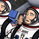17985 views
This is a replica of TTM Wave A B C.

The ABC Waves are comprised of various moving averages and oscillators ( MACD ) used to visualize the overall strength and direction of a given market across multiple time frames.
The “A Wave” measures short term relative strength and direction of a market, the “C Wave” measures longer term strength and the “B Wave” plots the same for a medium time period.

Here is the link to the ACTUAL Indicator - http://members.simpleroptions.com/option...

Instruction -
Load the Indicator three times, Turn Off the Other two Waves. For eg., Wave A - Check / Wave B - Uncheck / Wave C - Uncheck. = This will plot Wave A.
```// Created by UCSGEARS on 8/30/2014
// Updated - 03/22/2014

study(title="UCS_TTM_Wave A & B & C", shorttitle="WAVE-A/B/C", precision = 2)

usewa = input(true, title = "Wave A", type=bool)
usewb = input(true, title = "Wave B", type=bool)
usewc = input(true, title = "Wave C", type=bool)

// WAVE CALC
// Wave A
fastMA1 = usewa ? ema(close, 8) : na
slowMA1 = usewa ? ema(close, 34) : na
macd1 =  usewa ? fastMA1 - slowMA1 : na
signal1 =  usewa ? ema(macd1, 34) : na
hist1 =  usewa ? macd1 - signal1 : na

fastMA2 = usewa ? ema(close, 8) : na
slowMA2 = usewa ? ema(close, 55) : na
macd2 = usewa ? fastMA2 - slowMA2 : na
signal2 = usewa ? ema(macd2, 55) : na
hist2 = usewa ? macd2 - signal2 : na

// Wave B
fastMA3 = usewb ? ema(close, 8) : na
slowMA3 = usewb ? ema(close, 89) : na
macd3 = usewb ? fastMA3 - slowMA3 : na
signal3 = usewb ? ema(macd3, 89) : na
hist3 = usewb ? macd3 - signal3 : na

fastMA4 = usewb ? ema(close, 8) : na
slowMA4 = usewb ? ema(close, 144) : na
macd4 = usewb ? fastMA4 - slowMA4 : na
signal4 = usewb ? ema(macd4, 144) : na
hist4 = usewb ? macd4 - signal4 : na

// Wave C
fastMA5 = usewc ? ema(close, 8) : na
slowMA5 = usewc ? ema(close, 233) : na
macd5 = usewc ? fastMA5 - slowMA5 : na
signal5 = usewc ? ema(macd5, 233) : na
hist5 = usewc ? macd5 - signal5 : na

fastMA6 = usewc ? ema(close, 8) : na
slowMA6 = usewc ? ema(close, 377) : na
macd6 = usewc ? fastMA6 - slowMA6 : na

// PLOTs
plot(macd6, color=#FF0000, style=histogram, title="Wave C1", linewidth=3)
plot(hist5, color=#FF8C00, style=histogram, title="Wave C2", linewidth=3)

plot(hist4, color=#FF00FF, style=histogram, title="Wave B1", linewidth=3)
plot(hist3, color=#0000FF, style=histogram, title="Wave B2", linewidth=3)

plot(hist2, color=#008000, style=histogram, title="Wave A1", linewidth=3)
plot(hist1, color=#DAA520, style=histogram, title="Wave A2", linewidth=3)

hline(0, color=black, title = "Zero Line", linewidth = 2, linestyle = solid)
```